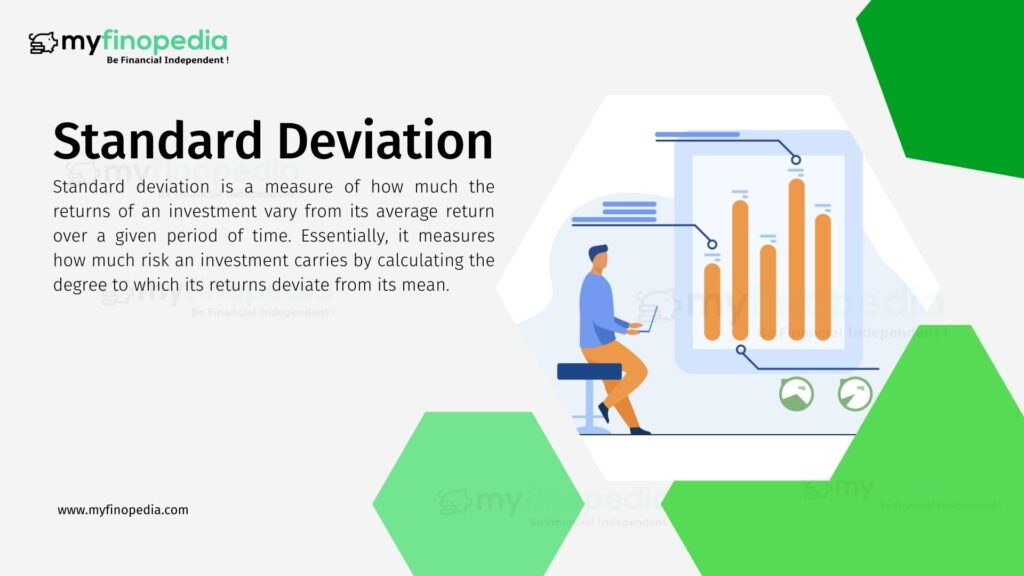# Standard DeviationWays To Measure Risk in Mutual Funds: Standard Deviation

One of the most common ways to measure risk in mutual funds is through a statistical measurement known as standard deviation. Standard deviation is a measure of how much the returns of an investment vary from its average return over a given period of time. Essentially, it measures how much risk an investment carries by calculating the degree to which its returns deviate from its mean.

To calculate the standard deviation of a mutual fund, one must first calculate the average return of the fund over a certain period of time. This is typically done using historical data, such as the fund’s performance over the past year or several years. Once the average return is calculated, the individual returns for each period are subtracted from the average, squared, and then summed up. This sum is then divided by the number of periods minus one, and the square root of this number is taken to arrive at the standard deviation.

For example, if a mutual fund had an average annual return of 8% over the past five years, and its individual returns for those years were 5%, 7%, 11%, 4%, and 9%, the calculation for standard deviation would be as follows:

The standard deviation for this mutual fund over the past five years would be 2.84%. This means that the returns of the fund varied, on average, by 2.84% from its mean return over the past five years. The higher the standard deviation, the greater the risk associated with the investment as it suggests that the investment’s returns have been more volatile and unpredictable. Investors who are risk-averse may prefer investments with lower standard deviations, as they provide a more stable and predictable return. On the other hand, investors who are willing to take on more risk may be more interested in investments with higher standard deviations, as they offer the potential for greater returns, but also come with greater risk.

The standard deviation for this mutual fund over the past five years would be 2.84%. This means that the returns of the fund varied, on average, by 2.84% from its mean return over the past five years. The higher the standard deviation, the greater the risk associated with the investment as it suggests that the investment’s returns have been more volatile and unpredictable. Investors who are risk-averse may prefer investments with lower standard deviations, as they provide a more stable and predictable return. On the other hand, investors who are willing to take on more risk may be more interested in investments with higher standard deviations, as they offer the potential for greater returns, but also come with greater risk.